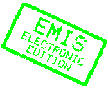MATHEMATICA BOHEMICA, Vol. 123, No. 4, pp. 411-418 (1998)

# Disjoint sequences in Boolean algebras

## Jan Jakubik

Jan Jakubik, Matematicky ustav SAV, Gresakova 6, 040 01 Kosice, Slovakia, e-mail: musavke@mail.saske.skAbstract: We deal with the system ${\operatorname{Conv}} B$ of all sequential convergences on a Boolean algebra $B$. We prove that if $\alpha$ is a sequential convergence on $B$ which is generated by a set of disjoint sequences and if $\beta$ is any element of ${\operatorname{Conv}} B$, then the join $\alpha\vee\beta$ exists in the partially ordered set ${\operatorname{Conv}} B$. Further we show that each interval of ${\operatorname{Conv}} B$ is a Brouwerian lattice.

Keywords: Boolean algebra, sequential convergence, disjoint sequence

Classification (MSC2000): 06E99, 11B99

Full text of the article:

[Previous Article] [Next Article] [Contents of this Number] [Journals Homepage]
© 2000 ELibM for the EMIS Electronic Edition